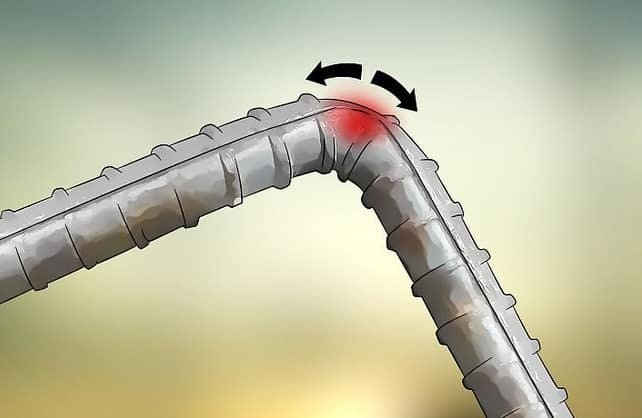# How To Calculate Bend Deduction Length Of Bar

How To Calculate Bend Deduction Length Of Bar:

When we bend a steel bar, the length of the bar slightly increased due to stretching in the bending area (refer to below image). The expansion of length depends on the grade of steel and the degree of bend. The length increases with the increase of bending degree and decreases with the higher grade steel. (Fe250, Fe450, Fe500)In this article, I will discuss how to calculate the bend deduction length for bars.

The formulae for bend deduction are

1. For 45° bend = 1 x d2. For 90° bend = 2 x d3. For 135° Bend = 3 x d4. For 180° bend = 4 x dWhere d = diameter of the bar.

### 4 thoughts on “How To Calculate Bend Deduction Length Of Bar”

1. Steel fixer Foreman

Reply
2. very helpful thanks

Reply
3. Where it is given the values of bend dedcution

Reply
4. There are the formulas given but there is not mentioned the number of bends. Pls make sure full formula should be given. Like at 90 degree
2*d*number of bends

Reply
error: Content is protected !!